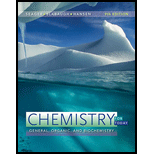# Balance the following equation. How many electrons will be transferred to NADH ? CH 4 + O 2 + _ _ _ NAD + → CO 2 + _ _ _ NADH + _ _ _ H +### Chemistry for Today: General, Orga...

9th Edition
Spencer L. Seager + 2 others
Publisher: Cengage Learning
ISBN: 9781305960060

#### Solutions

Chapter
Section### Chemistry for Today: General, Orga...

9th Edition
Spencer L. Seager + 2 others
Publisher: Cengage Learning
ISBN: 9781305960060
Chapter 22, Problem 22.54E
Textbook Problem
1 views

## Balance the following equation. How many electrons will be transferred to NADH ? CH 4 + O 2 + _ _ _ NAD + → CO 2 + _ _ _ NADH + _ _ _ H +

Interpretation Introduction

Interpretation:

The equation CH4+O2+___NAD+CO2+___NADH+___H+ is to be balanced and the number of electrons transferred to NADH is to be predicted.

Concept introduction:

The full form of NAD+ coenzyme is nicotinamide adenine dinucleotide. It is a coenzyme in which two nucleotides are joined together by a phosphate groups. It carries electrons from citric acid cycle to the electron transport chain.

### Explanation of Solution

The unbalanced equation is shown below.

The number of carbon and oxygen atoms on the left hand side and right hand side of the equation are equal but the number of hydrogen atoms and NAD is not equal. The number of hydrogen atoms on left hand side and right hand side is 4 and 2, respectively. The balanced equation is shown below

### Still sussing out bartleby?

Check out a sample textbook solution.

See a sample solution

#### The Solution to Your Study Problems

Bartleby provides explanations to thousands of textbook problems written by our experts, many with advanced degrees!

Get Started

Find more solutions based on key concepts
How might nutritional genomics influence health care in the future?

Understanding Nutrition (MindTap Course List)

What is the maximum number of bonds that a carbon atom can form?

Biology: The Dynamic Science (MindTap Course List)

The scientific discipline that uses ecological theory to study design, manage, and evaluate productive agricult...

Nutrition: Concepts and Controversies - Standalone book (MindTap Course List)

How does the equilibrium theory of tides differ from the dynamic theory?

Oceanography: An Invitation To Marine Science, Loose-leaf Versin

A model of a red blood cell portrays the cell as a capacitor with two spherical plates. It is a positively char...

Physics for Scientists and Engineers, Technology Update (No access codes included)